# HSSlive: Plus One & Plus Two Notes & Solutions for Kerala State Board

## AP Board Class 7 Maths Chapter 8 Exponents and Powers InText Questions Textbook Solutions PDF: Download Andhra Pradesh Board STD 7th Maths Chapter 8 Exponents and Powers InText Questions Book AnswersAP Board Class 7 Maths Chapter 8 Exponents and Powers InText Questions Textbook Solutions PDF: Download Andhra Pradesh Board STD 7th Maths Chapter 8 Exponents and Powers InText Questions Book Answers

## Andhra Pradesh State Board Class 7th Maths Chapter 8 Exponents and Powers InText Questions Books Solutions

 Board AP Board Materials Textbook Solutions/Guide Format DOC/PDF Class 7th Subject Maths Chapters Maths Chapter 8 Exponents and Powers InText Questions Provider Hsslive

2. Click on the Andhra Pradesh Board Class 7th Maths Chapter 8 Exponents and Powers InText Questions Answers.
3. Look for your Andhra Pradesh Board STD 7th Maths Chapter 8 Exponents and Powers InText Questions Textbooks PDF.
4. Now download or read the Andhra Pradesh Board Class 7th Maths Chapter 8 Exponents and Powers InText Questions Textbook Solutions for PDF Free.

## AP Board Class 7th Maths Chapter 8 Exponents and Powers InText Questions Textbooks Solutions with Answer PDF Download

Find below the list of all AP Board Class 7th Maths Chapter 8 Exponents and Powers InText Questions Textbook Solutions for PDF’s for you to download and prepare for the upcoming exams:

Question 1.
Write the following in exponential form by using 10 as the base number :
(i) 10,00,00,000
(ii) 100,00,00,000

 Number Expanded form Exponential (i) 10,00,00,000 10 × 10 × 10 × 10 × 10 × 10 × 10 × 10 108 (ii) 100,00,00,000 10 × 10 × 10 × 10 × 10 × 10 × 10 × 10 × 10 109

Let’s Explore [Page No. 29]

Question 1.
Observe and complete the following table. First one is done for you.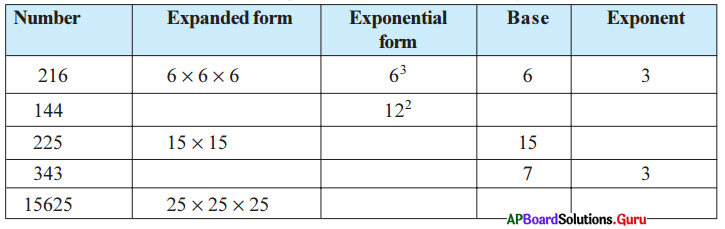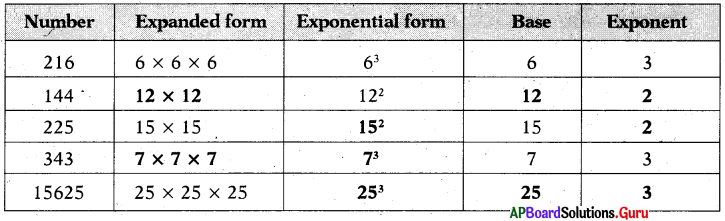Question 2.
Write the following numbers in exponential form. Also state the base, exponent and how to read. .
(i) 16
(ii) 49
(iii) 512
(iv) 243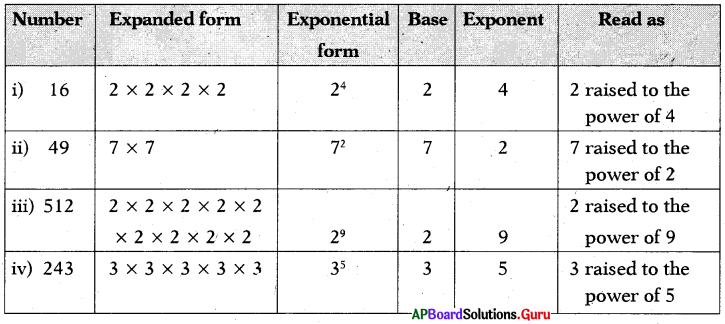Question 3.
Compute the following and write the greater one.
(i) 43 or 34
43 = 4 × 4 × 4 = 64
34 = 3 × 3 × 3 × 3 = 81 is greater.
64 < 81 (or) 81 > 64
So, 43 < 34 (or) 34 > 43

(ii) 53 or 35
53 = 5 × 5 × 5 = 125
35 = 3 × 3 × 3 × 3 × 3 = 243 is greater.
125 < 243 (or) 243 > 125
So, 53 < 35 (or) 35 > 53

Question 4.
32 = 3 × 3 = 9
23 = 2 × 2 × 2 = 8
9 ≠ 8
32 ≠ 23
So, ab ≠ ba unless a = b

Check Your Progress [Page No. 30]

Express the following number in exponential form using prime factorisation:
(i) 432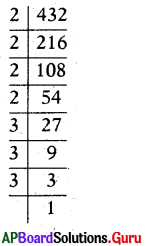432 = 2 × 216
= 2 × 2 × 108
= 2 × 2 × 2 × 54
= 2 × 2 × 2 × 2 × 27
= 2 × 2 × 2 × 2 × 3 × 9
= 2 × 2 × 2 × 2 × 3 × 3 × 3
∴ 432 = 24 × 33

(ii) 1296
1296 = 2 × 648
= 2 × 2 × 324
= 2 × 2 × 2 × 162
= 2 × 2 × 2 × 2 × 81
= 2 × 2 × 2 × 2 × 3 × 27
= 2 × 2 × 2 × 2 × 3 × 3 × 9
= 2 × 2 × 2 × 2 × 3 × 3 × 3 × 3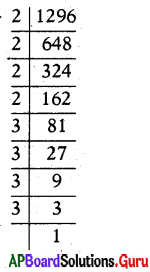∴ 1296 = 24 × 34

(iii) 729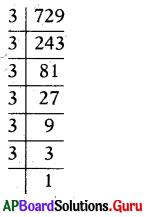729 = 3 × 243
= 3 × 3 × 81
= 3 × 3 × 3 × 27
= 3 × 3 × 3 × 3 × 9
= 3 × 3 × 3 × 3 × 3 × 3
729 = 36

(iv) 1600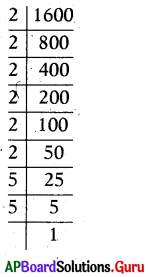1600 = 2 × 800
= 2 × 2 × 400
= 2 × 2 × 2 × 200
= 2 × 2 × 2 × 2 × 100
= 2 × 2 × 2 × 2 × 2 × 50
= 2 × 2 × 2 × 2 × 2 × 2 × 25
= 2 × 2 × 2 × 2 × 2 × 2 × 5 × 5
1600 = 26 × 52

Let’s Explore [Page No. 32]

Question 1.
Write the appropriate number in place of ▢ in the following.
Let ‘b’ be any non-zero integer.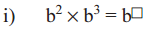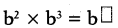= b2 × b3
= b × b × b × b × b = b5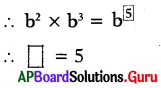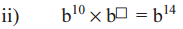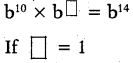then b10 × b1 = b14
b10+1 = b14
b11 ≠ b14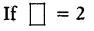then b10 × b2 = b14
b10+2 = b14
b12 ≠ b14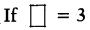then b10 × b3 = b14
b10+3 = b14
b13 ≠ b14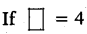then b10 × b4 = b14
b10+4 = b14
∴ b14 = b14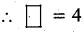Question 2.
Simplify the following using the formula am × an = am+n
(i) 57 × 54
57 × 54
We know that am × an = am+n;
57 × 54 = 57+4 = 511
∴ 57 × 54 = 511

(ii) p3 × p2
p3 × p2
We know that am × an = am+n
p3 × p2 = p3+2 = p5
∴ p3 × p2 = p5

(iii) (-4)10 × (-4)3 × (-4)2
(-4)10 × (-4)3 × (-4)2
We know that am × an = am+n
(- 4)10+3+2 = (- 4)15
∴ (-4)10 × (-4)3 × (-4)2 = (- 4)15

Let’s Explore [Page No. 33]

Question 1.
Write the following in exponential form using the formula (am)n = amn.
(i) (62)4
(62)4
We know (am)n = amn
(62)4 = 62×4 = 68
∴ (62)4 = 68

(ii) (22)100
(22)100
We know (am)n = amn
(22)100 = 22×100 = 2200
∴ (22)100 = 2200

(iii) (206)2
(206)2
We know (am)n = amn
(206)2 = 206×2 = 2012
∴ (206)2 = 2012

(iv) [(-10)3]5
[(-10)3]5
We know (am)n = amn
[(-10)3]5 =(-10)3×5 = (-10)15
∴ [(- 10)3]5 = (- 10)15

Check Your Progress [Page No. 34]

Simplify the following by using the law , am × bm = (ab)m.
(i) 76 × 36
76 × 36
We know, am × bm = (ab)m
76 × 36 = (7 × 3)6 = (21)6
∴ 76 × 36 = 216

(ii) (3 × 5)4
(3 × 5)4
We know, (ab)m = am × bm
(3 × 5)4 = 34 × 54
∴ (3 × 5)4 = 34 × 54

(iii) a4 × b4
a4 × b4
We know, am × bm = (ab)m
a4 × b4 = (a . b)4
∴ a4 × b4 = (a . b)4

(iv) 32 × a2
32 × a2
We know, am × bm = (ab)m
32 × a2 = (3 × a)2 = (3a)2
∴ 32 × a2 = (3a)2

Let’s Explore [Page No. 37]

Question 1.
Simplify and write In the form of am-n Or 1𝐚𝐧𝐦
(i) 108104
We Know 108104 = am-n
108104 = 108-4 = 104
108104 = 104

(ii) (7)13(7)10
(7)13(7)10
We Know 𝑎𝑚𝑎𝑛 = am-n (m > n)
(7)13(7)10 = (-7)13-10
= (-7)3 = -7 × -7 × -7 = – 343
(7)13(7)10 = – 343

(iii) 125128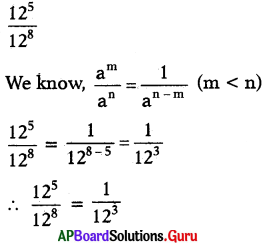(iv) 3437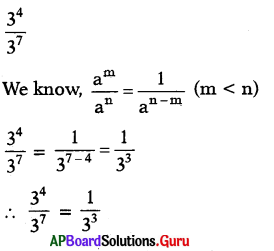Question 2.
Fill the appropriate number In the box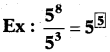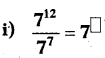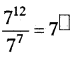We Know 𝑎𝑚𝑎𝑛 = am-n (m > n)
71277 = 712-7 = 75
∴ 71277 = 75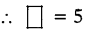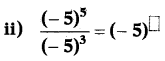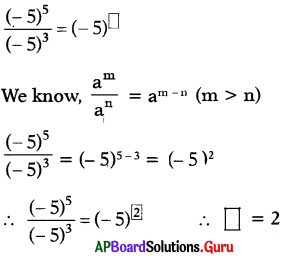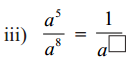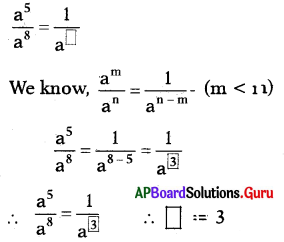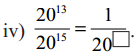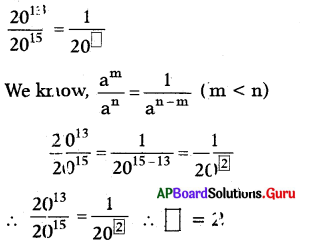Question 3.
Simplify the following :
(i) 6868
6868
We Know 𝑎𝑚𝑎𝑛 = am-n
6868 = 68-8 = 60 = 1 (∵ a0 = 1)

(ii) 𝑡10𝑡10
𝑡10𝑡10
We Know 𝑎𝑚𝑎𝑛 = am-n
𝑡10𝑡10 = t10-10 = t0 = 1 (∵ a0 = 1)

(iii) 127127
127127
We Know 𝑎𝑚𝑎𝑛 = am-n
127127 = 127-7 = 120 = 1 (∵ a0 = 1)

(iv) 𝑝5𝑝5
𝑝5𝑝5
We Know 𝑎𝑚𝑎𝑛 = am-n
𝑝5𝑝5 = p10-10 = p0 = 1 (∵ a0 = 1)

Check Your Progress [Page No. 38]

Question 1.
Complete the following boxes .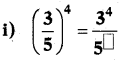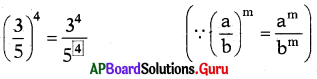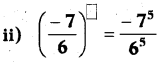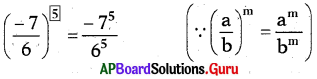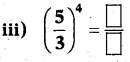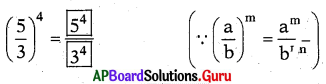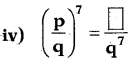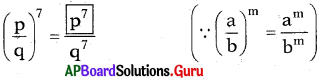Check Your Progress [Page No. 39]

Question 1.
Express the following in exponential form.
(i) 27125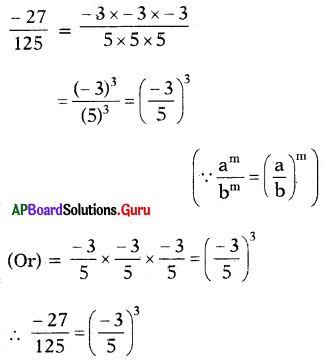(ii) 32243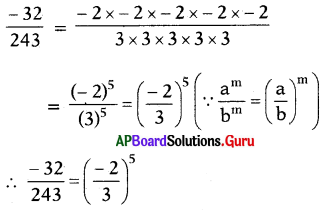(iii) 1251000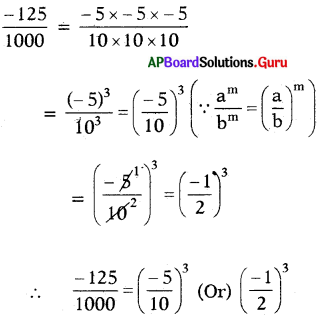(iv) 1625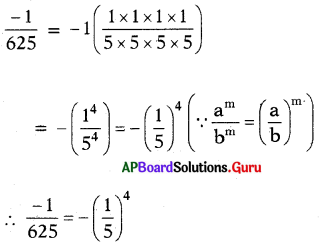Let’s Think [Page No. 39]

Question 1.
Deekshltha and Harsha computed 4(3)2 in different ways.
Deeksbitha did it like this
4(3)2 = (4 × 3)2
= 122
= 144

Harsha did it like this
4(3)2 = 4 × (3 × 3)
= 4 × 9
= 36
Who has done the problem Incorrectly?
Discuss the reason for the mistake with your friends.
In 4(3)2 square only belongs to 3, but not 4.
So, Deekshitha did wrong and Harsha did correct.

Let’s Do Activity [Page No. 40]

Finding the pair : Divide the classroom into two groups. Each group has a set of cards. Each student of group 1 has to pair with one suitable student of group 2 by stating in the reason.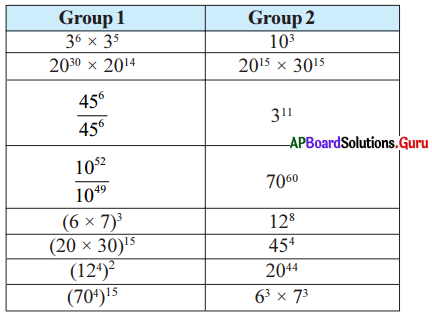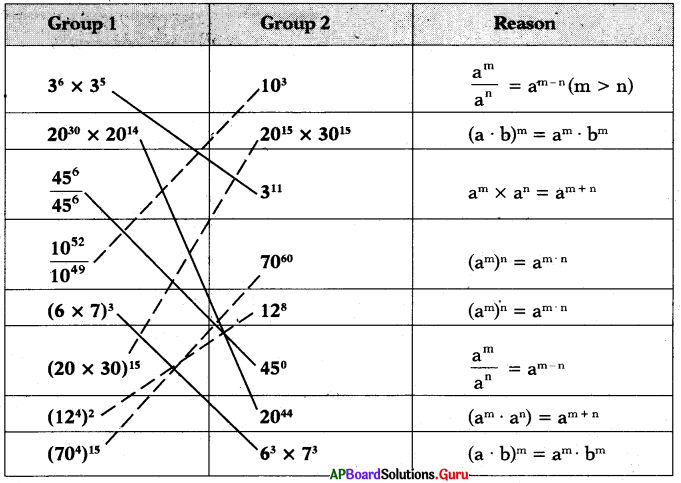Note : This activity can be extended till all the children in the class are familiarised with the laws of exponents.

Project Work [Page No. 42]

Collect the annual income of 5 families in your location by observing their ration card and rounded into the nearest thousand / Lakh and express in the exponential form. One done for you.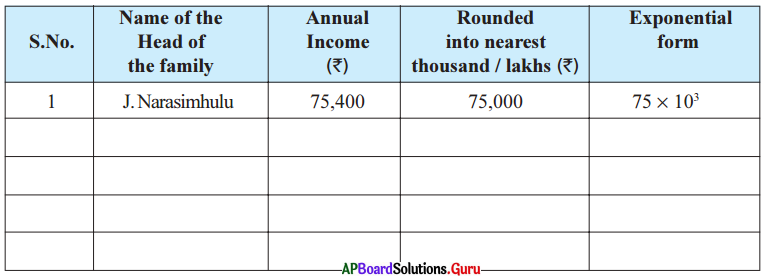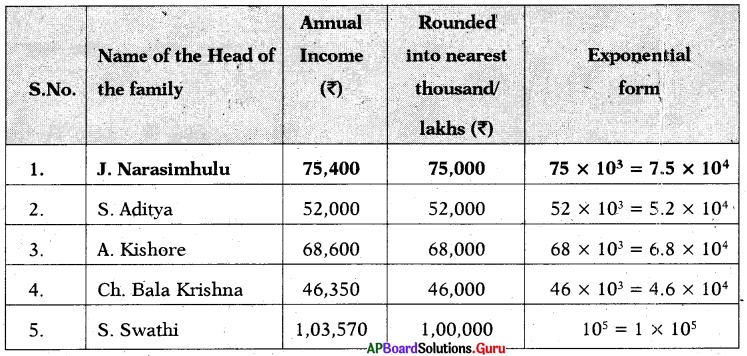Examples:

Question 1.
Which one is greater 82 or 28? Justify.
82 = 8 × 8 = 64
28 = 2 × 2 × 2 × 2 × 2 × 2 × 2 × 2 = 256
256 >64
Therefore, 28 > 82.

Question 2.
Simplify the following using the formula am × an = am+n
(i) (- 5)7 × (- 5)4
(- 5)7 × (- 5)4 = (- 5)7+4
(∵ am × an = am+n)
= (- 5)11
∴ (- 5)7 × (- 5)4 = (- 5)11

(ii) 33 × 32 × 34
33 × 32 × 34 = 33+2+4
(∵ am × an = am+n)
= 39
∴ 33 × 32 × 34 = 39

Question 3.
Simplify the following using the formula (am)n = amn
(i) (83)4
(83)4 = 83×4
= 812
∴ (83)4 = 812

(ii) [(-11)5]2
[(-11)5]2 = (- 11)5×2
= (- 11)10
∴ [(-11)5]2 = (- 11)10

(iii) (750)2
(750)2= 750×2 = 7100
∴ (750)2 = 7100

Question 4.
Simplify the following using the expo-nential law am × bm = (ab)m
(i) 52 × 32
52 × 32 = (5 × 3)2 [∵ am × bm = (ab)m]

(ii) p3 × q3
p3 × q3 = (p × q)3

(iii) (7 × 8)4
(7 × 8)4 = 74 × 84 [∵ (ab)m = am × bm]

Question 5.
Simplify the following and write in the form of 𝑎𝑚𝑎𝑛 = am-n or 𝑎𝑚𝑎𝑛=1𝑎𝑛𝑚
(i) 2923
2923 = 29-3 [∵ 𝑎𝑚𝑎𝑛 = am-n]
= 26

(ii) (9)11(9)7
(9)11(9)7 = (- 9)11-7 = (-9)4

(iii) 710713
710713=171310
[∵ 𝑎𝑚𝑎𝑛=1𝑎𝑛𝑚]
173

(iv) 6265
6265=1652=163

Question 6.
Simplify the following by using formula 𝑎𝑚𝑏𝑚=(𝑎𝑏)𝑚.
(i) 5323
5323=(52)3 [∵ 𝑎𝑚𝑏𝑚=(𝑎𝑏)𝑚]

(ii) (85)4
(85)4=8454
[∵ 𝑎𝑚𝑏𝑚=(𝑎𝑏)𝑚]

Question 7.
Evaluate:
(1)4, (1)5, (1)7, (- 1)2, (- 1)3, (- 1)4, (- 1)5
(1)4 = 1 × 1 × 1 × 1 = 1
(1)5 = 1 × 1 × 1 × 1 × 1 = 1
(1)7 = 1 × 1 × 1 × 1 × 1 × 1 × 1 = 1
(- 1)2= (-1) × (-1) = 1
(- 1)3 = (-1) × (1) × (-1) = – 1
(- 1)4 =(-1) × (-1) × (-1) × (-1) = 1
(- 1)5 = (-1) × (-1) × (-1) × (-1) × (- 1) = – 1

From the above illustrations,
(i) It raised. to any power is 1.
(ii) (-1) raised to even power is (- 1) and
(-1) raised to an odd power is (-1).

Thus (- 1)m = 1 if ’m’ is even
(- 1)m 1 if ‘m’ is odd

Question 8.
Express  In exponential form.
-8 = (-2) × (-2) × (-2) = (-2)3
27 = 3 × 3 × 3 = (3)3
∴ 827:(2)333=(23)3

Question 9.
Abhllash computed a3. a2 as a6. Is it correct?
Abhilash has done it incorrectly.
Bcause a3. a2 = a3+2 = a5 [∵ am . an = amn]
Therefore, a3. a2 = a5 is correct answer.

Question 10.
Riyaz computed 𝑎8𝑎2 as a4. Has he done It correctly? Justify your answer.
Riyaz has done it incorrectly.
Because 𝑎8𝑎2 = a8-2
= a6 [∵ 𝑎𝑚𝑎𝑛 = am-n]
∴ 𝑎8𝑎2 = a6 is correct answer.

Question 11.
Write the following into standard form.
(i) 7465
7465 = 7.465 × 1000 (Decimal is shifted three places to the left)
= 7.465 × 103

(ii) The height of Mount Everest is 8848 m.
The height of Mount Everest
= 8848 m
= 8.848 × 1000 m (Decimal is shifted three places to the left)
= 8.848 × 103m

(iii) The distance from the Sun to Earth is 149,600,000,000 m.
The distance from the Sun to Earth
= 149,600,000,000 m
= 1.496 × 100000000000 m
= 1.496 × 1011 m

Reasoning Corner [Page No. 45: Odd one out in numbers]

In each of the following questions, there are 4 numbers. Three of them are similar in a certain way but one is not like the other three. One has to identify the similarity and then strike the odd one out as answer option.
The number can be odd/ even /consecutive, prime numbers, multiple of some number, single, square or cubes of different numbers, plus/minus of some other number or combinations of any mathematical calculation.
Question 1.
(a) 12
(b) 25
(c) 37
(d) 49
(c) 37

Hint:
Prime number

Question 2.
(a) 13
(b) 63
(c) 83
(d) 43
(b) 63

Hint:
Not a prime number

Question 3.
(a) 21
(b) 49
(c) 56
(d) 36
(d) 36

Hint:
Not divisible by 7

Question 4.
(a) 112
(b) 256
(c) 118
(d) 214
(b) 256

Hint:
Square number

Question 5.
(a) 42
(b) 21
(c) 84
(d) 35
(d) 35

Hint:
Not divisible by 3

Question 6.
(a) 11
(b) 13
(c) 15
(d) 17
(c) 15

Hint:
Not a prime number

Question 7.
(a) 10
(b) 11
(c) 15
(d) 16
(b) 11

Hint:
Prime number

Question 8.
(a) 49
(b) 63
(c) 77
(d) 81
(d) 81

Hint:
Not divisible by 7

Question 9.
(a) 28
(b) 65
(c) 129
(d) 215
(a) 28

Hint:
Even number

Question 10.
(a) 51
(b) 144
(c) 64
(d) 121
(a) 51

Hint:
Not square number

Practice Questions [Page No. 46]

Question 1.
(a) 3
(b) 9
(c) 5
(d) 7
(b) 9

Explanation:
9 is composite number. The remaining numbers are primes.

Question 2.
(a) 6450
(b) 1776
(c) 2392
(d) 3815
(d) 3815

Explanation:
All others are even numbers.

Question 3.
(a) 24
(b) 48
(c) 42
(d) 12
(c) 42

Explanation:
12, 24 and 48 are multiples of 12.

Question 4.
(a) 616
(b) 252
(c) 311
(d) 707
(c) 311

Explanation:
616, 252 and 707 are Palindromes.

Question 5.
(a) 18
(b) 12
(c) 30
(d) 20
(d) 20

Explanation:
12, 18 and 30 are 3 (or) 6 multiples. But, 20 is not 3 (or) 6 multiple.

Question 6.
Find the odd one from the given
(a) 3730
(b) 6820
(c) 5568
(d) 4604
(a) 3730

Explanation:
3730, All others are divisible by 4.

Question 7.
(a) 2587
(b) 7628
(c) 8726
(d) 2867
(a) 2587

Explanation:
All others are formed by 2, 6, 7 and 8.

Question 8.
(a) 63
(b) 29
(c) 27
(d) 25
(d) 25

Explanation:
63, 29 and 27 are not squares. 25 only the square.

Question 9.
(a) 23
(b) 37
(c) 21
(d) 31
(c) 21

Explanation:
23,37 and 31 are prime numbers.
21 only the composite number.

Question 10.
(a) 18
(b) 9
(c) 21
(d) 7
(d) 7

Explanation:
18, 9, 21 are composite numbers.
7 only the prime number.

## Andhra Pradesh Board Class 7th Maths Chapter 8 Exponents and Powers InText Questions Textbooks for Exam Preparations

Andhra Pradesh Board Class 7th Maths Chapter 8 Exponents and Powers InText Questions Textbook Solutions can be of great help in your Andhra Pradesh Board Class 7th Maths Chapter 8 Exponents and Powers InText Questions exam preparation. The AP Board STD 7th Maths Chapter 8 Exponents and Powers InText Questions Textbooks study material, used with the English medium textbooks, can help you complete the entire Class 7th Maths Chapter 8 Exponents and Powers InText Questions Books State Board syllabus with maximum efficiency.

## FAQs Regarding Andhra Pradesh Board Class 7th Maths Chapter 8 Exponents and Powers InText Questions Textbook Solutions

#### Can we get a Andhra Pradesh State Board Book PDF for all Classes?

Yes you can get Andhra Pradesh Board Text Book PDF for all classes using the links provided in the above article.

## Important Terms

Andhra Pradesh Board Class 7th Maths Chapter 8 Exponents and Powers InText Questions, AP Board Class 7th Maths Chapter 8 Exponents and Powers InText Questions Textbooks, Andhra Pradesh State Board Class 7th Maths Chapter 8 Exponents and Powers InText Questions, Andhra Pradesh State Board Class 7th Maths Chapter 8 Exponents and Powers InText Questions Textbook solutions, AP Board Class 7th Maths Chapter 8 Exponents and Powers InText Questions Textbooks Solutions, Andhra Pradesh Board STD 7th Maths Chapter 8 Exponents and Powers InText Questions, AP Board STD 7th Maths Chapter 8 Exponents and Powers InText Questions Textbooks, Andhra Pradesh State Board STD 7th Maths Chapter 8 Exponents and Powers InText Questions, Andhra Pradesh State Board STD 7th Maths Chapter 8 Exponents and Powers InText Questions Textbook solutions, AP Board STD 7th Maths Chapter 8 Exponents and Powers InText Questions Textbooks Solutions,
Share: